# JavaScript Tutorial : Array and Array Methods

Array is like a drawer containing different things. You can open the drawer and pick things, count what is inside the drawer and even add to the contents there. That is basically what an array is all about.

It is a data structure composed of collection of elements which can be strings, numbers, or variables. These elements are assigned a key or what is known as array index. The elements are those items or data stored in the array. Array is useful in storing large data. Suppose you want to store details of hive users. Instead of assigning a variable for all the users which will enter hundreds of thousands variables, you can just store them in a single array. The names can then be accessed using the indexnumber.

### Creating an array

You can create an array in JavaScript as seen below

``````var blockchains = ['Hive', 'Ethereum', 'Eos', 'Steem'];
console.log(blockchains);
``````You can see how it looks like in the console. That is an array with values Hive, Ethereum, Eos, and Steem

### Accessing Array elements

You can access the Array elements by using the indexnumber and the index of the first element of an array is 0.

``````var blockchains = ['Hive', 'Ethereum', 'Eos', 'Steem'];
console.log(blockchains);
console.log(blockchains);
``````

This will return Ethereum in the search console. Hive will have the index 0, Ethereum index 1, Eos index 2, and Steem index 3.
You can also add to the elements of the array by using
array is 0.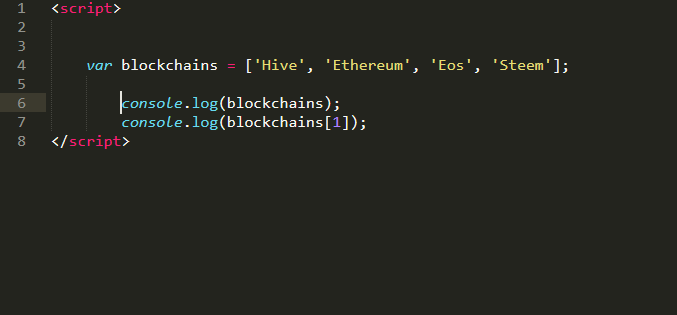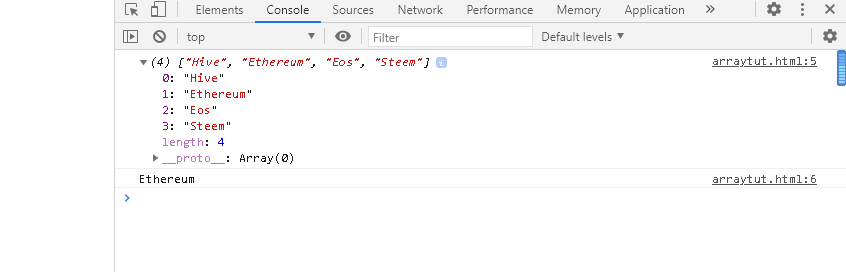``````var blockchains = ['Hive', 'Ethereum', 'Eos', 'Steem'];
blockchain = 2;
blockchain =’Bitcoin’
console.log(blockchains);
console.log(blockchains);
``````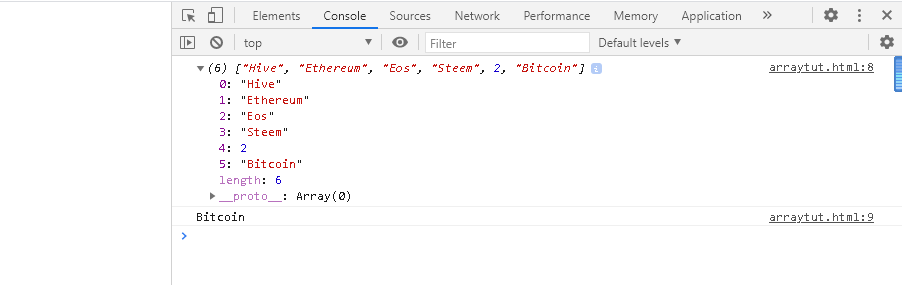The above code simply shows we are adding the number 2 to represent the array index 4 and bitcoin to represent array index 5. That is a simple way of adding elements to arrays.

### Array Length

You can know the number of elements in an array by using the Array.length code.

``````var blockchains = ['Hive', 'Ethereum', 'Eos', 'Steem'];
blockchain = 2;
blockchain =’Bitcoin’
console.log(blockchains);
console.log(‘The number of elements in the array is ’ + blockchains.length);

``````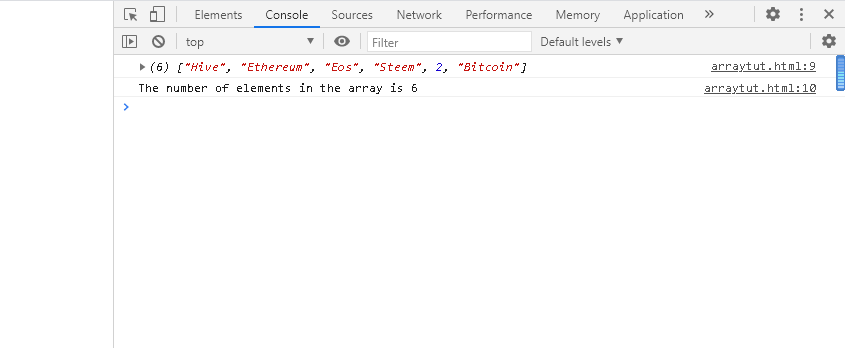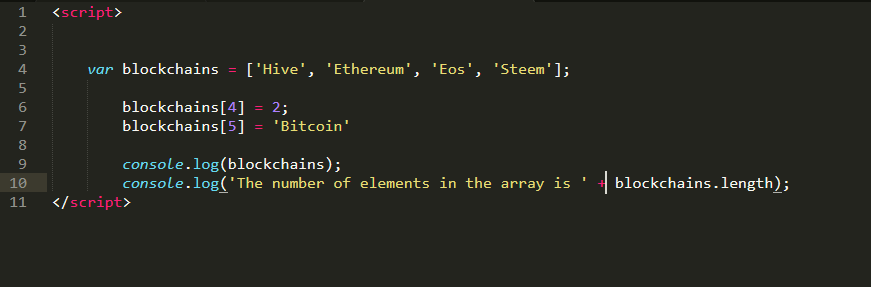This will tell us the number of elements and it starts counting from 1 not zero like the Array index. This mean the number of elements will be 6.

## Array Methods

We will look at some of the Array methods that can be applied to Arrays

### Concat

This joins two or more arrays together and then return a copy of the combined arrays

``````var blockchains = ['Hive', 'Ethereum', 'Eos', 'Steem'];
var coins = ['HBD', 'BNB', 'BTC'];
var founders = ['CZ', 'Dan', 'Lee', 'Larsen'];
var aggregation = blockchains.concat(coins, founders);

console.log(aggregation)
``````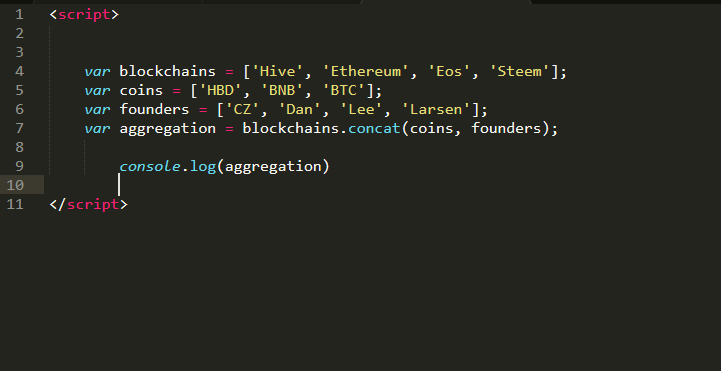You can see the result above. The arrays elements have been joined together.

### copyWithin

This copies array elements within an array into a specified position.

``````var blockchains = ['Hive', 'Ethereum', 'Eos', 'Steem'];
var coins = ['HBD', 'BNB', 'BTC'];
blockchains.copyWithin(0,1,2)

console.log(blockchains)
``````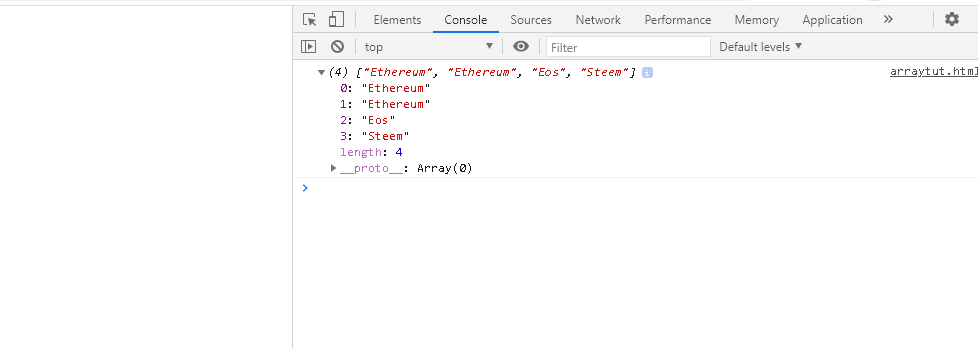The copyWithin(0,1,2) simply represents the parameters
* target the index position you want to copy to
* the index position to start copying from
* the index position to stop the copying
This mean I want the target position to be index 0 and I will start copying from index position 1 and stop at 2. That is why you see that Ethereum has overwritten Hive.

### Fill

The fill method will fill the Array elements with a static value specified as shown below

``````var blockchains = ['Hive', 'Ethereum', 'Eos', 'Steem'];
var coins = ['HBD', 'BNB', 'BTC'];

blockchains.fill('Graphene');
coins.fill('ETH', 0, 2);
console.log(blockchains);
console.log(coins);
``````fill() takes in 3 parameters
* The static value that will fill the array
* The start index to start filling the array
* The end index to stop filling the Array

### Filter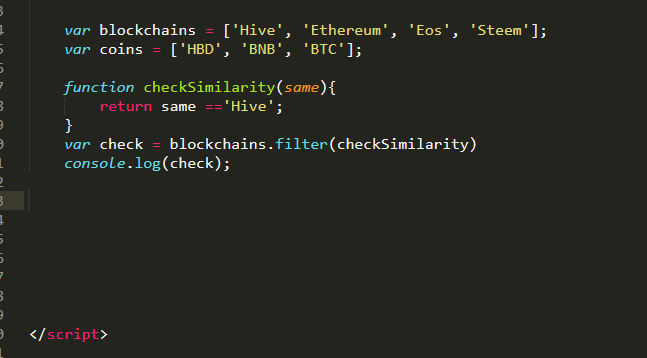This returns an array of all the elements that matches the Hive.

``````var blockchains = ['Hive', 'Ethereum', 'Eos', 'Steem'];
var coins = ['HBD', 'BNB', 'BTC'];

function checkSimilarity(same){
return same =='Hive';
}
var check = blockchains.filter(checkSimilarity)
console.log(check);

``````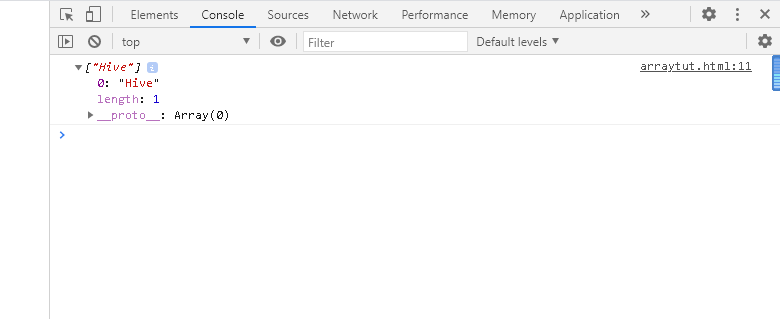### Find

Find returns the value of the Array element that matches the value Hive as specified in the checkSimilarity function.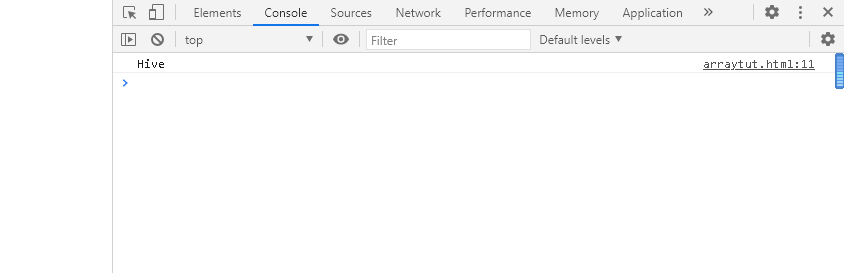``````var blockchains = ['Hive', 'Ethereum', 'Eos', 'Steem'];
var coins = ['HBD', 'BNB', 'BTC'];

function checkSimilarity(same){
return same =='Hive';
}
var check = blockchains.find(checkSimilarity)
console.log(check);
``````

### findIndex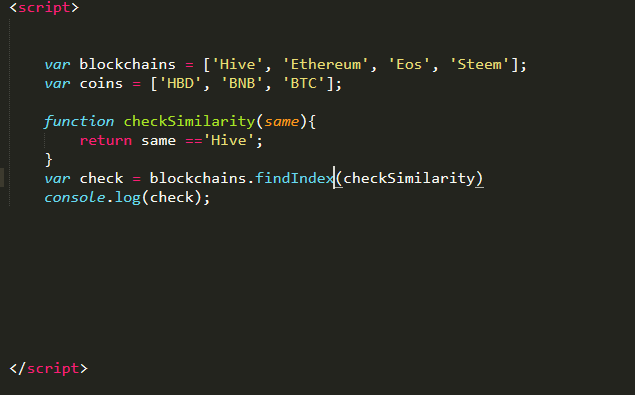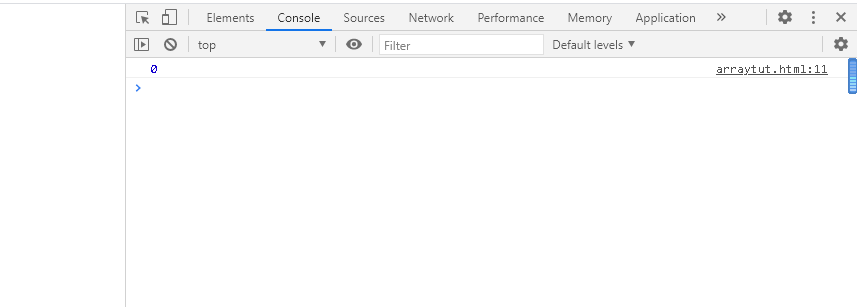This returns the index of the element in an array that matches ‘’Hive’’. This is important when you need to find the index of a particular element in an array. You can use this method for it.

``````var blockchains = ['Hive', 'Ethereum', 'Eos', 'Steem'];
var coins = ['HBD', 'BNB', 'BTC'];

function checkSimilarity(same){
return same =='Hive';
}
var check = blockchains.findIndex(checkSimilarity)
console.log(check);
``````

### forEach

The forEach assigns a function for each array elements. It runs through the array elements and run the function for each of them.
Below is a code that finds the sum of all the numbers in an array. You initialize the sum variable and assign value of 0. The add() function is simply saying add 0 to the element, then assign the result to the variable sum.
When we now say price.forEach(add);, this means 0+20 will run and assign 20 to the sum variable, then 20+30 will run and assign 50 to the sum variable, then 50+50 will run and assign 100 to the sum variable, then 100_60 will run and assign 160 to the sum variable then finally 160+110 will run and assign 270 to the sum variable which is displayed in our console.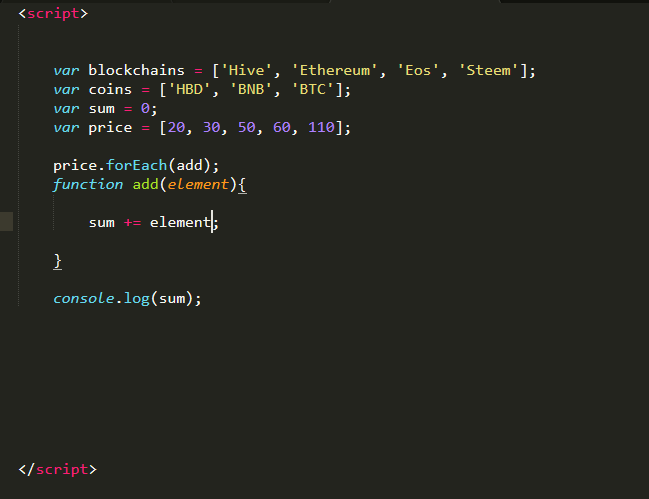You can modify the function to whatever to the array elements via the forEach method.

``````var blockchains = ['Hive', 'Ethereum', 'Eos', 'Steem'];
var coins = ['HBD', 'BNB', 'BTC'];
var sum = 0;
var price = [20, 30, 50, 60, 110];

sum += element;

}

console.log(sum);
``````

### From

The from() method creates an array from a string as shown below. The string I am a programmer will be turned into an array of length 17 elements.``````var blockchains = ['Hive', 'Ethereum', 'Eos', 'Steem'];
var coins = ['HBD', 'BNB', 'BTC'];

var myString = Array.from("I am a programmer");

console.log(myString);
``````

### Join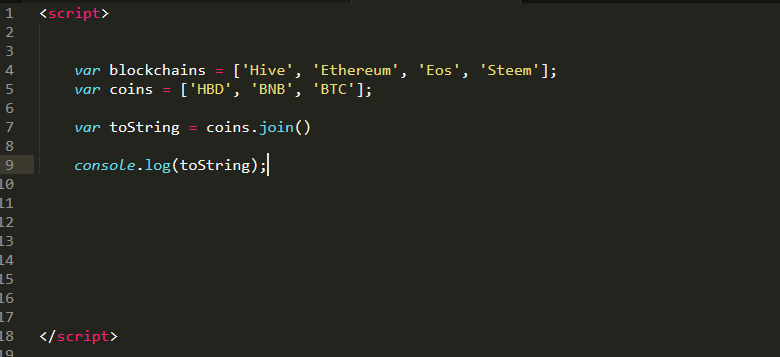This converts elements of an array into a string. It is like the opposite of from().``````var blockchains = ['Hive', 'Ethereum', 'Eos', 'Steem'];
var coins = ['HBD', 'BNB', 'BTC'];

var toString = coins.join()

console.log(toString);
``````

### Includes

The includes() method check if an array contains a specified element. It will return true if the element is present and false if it is absent.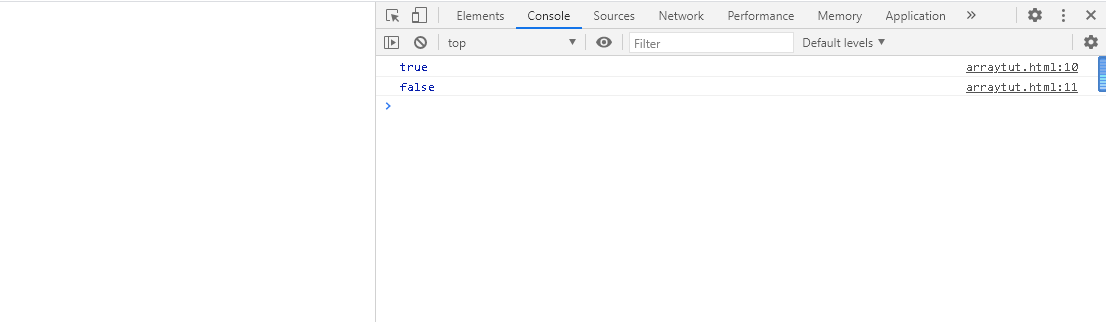``````var blockchains = ['Hive', 'Ethereum', 'Eos', 'Steem'];
var coins = ['HBD', 'BNB', 'BTC'];

var check = (blockchain.includes(‘Eos’));
var checkagain = (blockchain.includes(‘BCH’));

console.log(check);
console.log(checkagain);

``````

### MapMap creates a new array with the results of whatever function applied to the original array elements.
Below returns an array with the cuberoot of the numbers arrays. You can see that the original numbers arrays is not modified, instead, the result is assigned and displayed by the new array cubeRoot.

``````var blockchains = ['Hive', 'Ethereum', 'Eos', 'Steem'];
var coins = ['HBD', 'BNB', 'BTC'];
var numbers = [8, 27, 64, 125, 216]
var cubeRoot = numbers.map(Math.cbrt);

console.log(numbers);
console.log(cubeRoot);
``````

Other Array methods will be looked into in the next part
References
Ref 1 | Ref 2